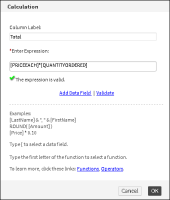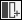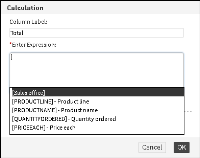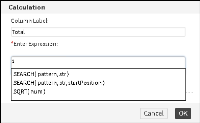Creating a computed column
When you create a computed column, you write an expression, which is a statement that indicates how to calculate the data. In the report in Figure 8‑1,
each value in the Total column is calculated by multiplying the value in the QUANTITYORDERED field with the value in the PRICEEACH field.
Figure 8‑2 shows the computed column, Total, using the following expression:
[PRICEEACH]*[QUANTITYORDERED]
When you refer to a data field in an expression, you must enclose the field name within brackets ([ ]). Field names are case‑sensitive.Figure 8‑2 An expression for the computed column
Report Studio supports typical mathematical operations, such as addition, subtraction, multiplication, and division. Computed columns, however, are not limited to mathematical calculations. Report Studio also supports many functions for manipulating date‑and‑time and string data. A function is a set of instructions that do something and return a result. For example, if a CustomerName field contains values with leading or trailing blank characters, you can remove the blank characters by using the TRIM( ) function as follows:
TRIM([CustomerName])
In this example, [CustomerName] is the input value, or argument, you supply to the TRIM( ) function to tell the function to trim the values in the CustomerName field.
Report Studio also enables you to create aggregate data for the values in a computed column. For descriptions of the supported functions, see Functions and operators.
How to create a computed column1 Select the column to the left of the new computed column you want to insert.
2 On the toolbar, choose New Computed Column.
3 In Calculation, type a name in Column Label for the computed column. The name you specify appears in the column header.
4 In Enter Expression, type the expression to calculate the values you want to display:To use a data field in the expression, type the left bracket ([), then select a field from the list that appears, as shown in Figure 8‑3.Figure 8‑3 Selecting a data field to use in an expression
The list shows only the fields in use in the BIRT design. It does not show all the fields in the data set. To use a field that is not in the list, you must add the field to the BIRT design.To use a function, type the first letter of the function, then select a function from the list that appears, as shown in Figure 8‑4. The functions indicate the arguments, if any, you need to supply.Figure 8‑4 Selecting a function to use in an expression
5 When you finish building the expression, choose Validate. If the expression is syntactically correct, the message, The Expression is valid appears. If the expression contains an error, a message that describes the error appears.
6 After you validate the expression, choose OK. The computed column appears in the BIRT design.